polarscatter

Scatter chart in polar coordinates

•Description

Vector and Matrix Data

example

polarscatter(theta,rho) plots theta versus rho and displays a circular marker at each data point. theta and rho must be vectors of the same length. You must specify theta in radians.

• To plot one set of points, specify theta and rho as vectors of equal length.

• To plot multiple sets of points within the same polar axes, specify at least one of theta or rho as a matrix.

polarscatter(theta,rho,sz) sets the marker sizes, where sz specifies the area of each marker in points squared. To draw all the markers with the same size, specify sz as a scalar. To draw the markers with different sizes, as a vector or a matrix.

example

polarscatter(theta,rho,sz,c) specifies the marker colors. You can specify one color for all the markers, or you can vary the color. For example, you can plot all red circles by specifying c as 'red'.

polarscatter(___,mkr) sets the marker symbol. For example, '+' displays cross markers. Specify the marker symbol after any of the input argument combinations in the previous syntaxes.

example

polarscatter(___,'filled') fills the marker interiors.

Table Data

example

polarscatter(tbl,thetavar,rhovar) plots the variables thetavar and rhovar from the table tbl. To plot one data set, specify one variable for thetavar and one variable for rhovar. To plot multiple data sets, specify multiple variables for thetavar, rhovar, or both. If both arguments specify multiple variables, they must specify the same number of variables. (Since R2021b)

example

polarscatter(tbl,thetavar,rhovar,'filled') plots the specified variables from the table with filled circles. (Since R2021b)

polarscatter(pax,___) plots into the polar axes specified by pax instead of into the current axes.

polarscatter(___,Name,Value) modifies the appearance of the scatter plot using one or more name-value pair arguments. For example, you can use semi-transparent markers by specifying 'FaceAlpha' and a scalar value between 0 and 1.

example

ps = polarscatter(___) returns the Scatter object or an array of Scatter objects.. Use ps to modify the appearance of the Scatter object after it is created. For a list of properties, see Scatter Properties.

Examples

collapse all

Create a scatter chart in polar coordinates.

th = pi/4:pi/4:2*pi;
r = [19 6 12 18 16 11 15 15];
polarscatter(th,r)Create a scatter chart that uses filled markers by specifying the optional input argument, 'filled'. Set the marker size to 75 points squared.

th = linspace(0,2*pi,20);
r = rand(1,20);
sz = 75;
polarscatter(th,r,sz,'filled')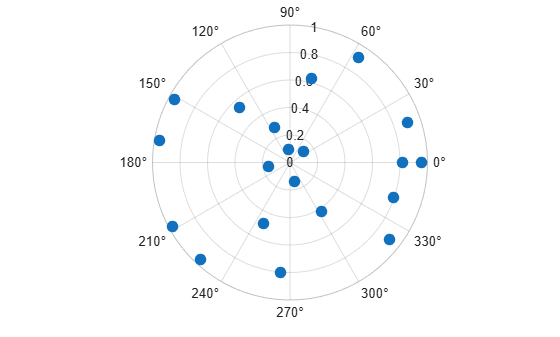Create a scatter chart with markers of varying sizes and colors. Specify the optional size and color input arguments as vectors. Use unique values in the color vector to specify the different colors you want. The values map to colors in the colormap.

th = pi/4:pi/4:2*pi;
r = [19 6 12 18 16 11 15 15];
sz = 100*[6 15 20 3 15 3 6 40];
c = [1 2 2 2 1 1 2 1];
polarscatter(th,r,sz,c,'filled','MarkerFaceAlpha',.5)Create data where the angle values are in degrees. Since polarscatter requires angle values in radians, convert the values to radians before plotting using deg2rad.

th = linspace(0,360,50);
r = 0.005*th/10;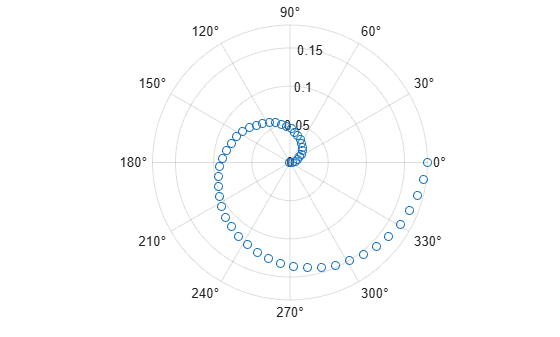Combine two scatter charts in the same polar axes using the hold command. Add a legend with a description of each chart.

th = pi/6:pi/6:2*pi;
r1 = rand(12,1);
polarscatter(th,r1,'filled')

hold on
r2 = rand(12,1);
polarscatter(th,r2,'filled')
hold off

legend('Series A','Series B')Create a scatter chart and assign the scatter object to the variable ps.

th = pi/6:pi/6:2*pi;
r = rand(12,1);
ps = polarscatter(th,r,'filled')ps =
Scatter with properties:

ThetaData: [0.5236 1.0472 1.5708 2.0944 2.6180 3.1416 3.6652 ... ]
RData: [0.8147 0.9058 0.1270 0.9134 0.6324 0.0975 0.2785 ... ]
ZData: [1x0 double]
SizeData: 36
CData: [0 0.4470 0.7410]
Marker: 'o'
MarkerEdgeColor: 'none'
MarkerFaceColor: 'flat'
LineWidth: 0.5000

Show all properties

Use ps to modify properties of the scatter object after it is created.

ps.Marker = 'square';
ps.SizeData = 200;
ps.MarkerFaceColor = 'red';
ps.MarkerFaceAlpha = .5;Since R2021b

A convenient way to plot data from a table is to pass the table to the polarscatter function and specify the variables you want to plot. For example, create a table with three variables, and plot the 'Th' and 'R1' variables.

% Create a table of random numbers
Th = linspace(0,2*pi,50)';
R1 = randi([0 10],50,1);
R2 = randi([20 30],50,1);
tbl = table(Th,R1,R2);

% Create polar scatter chart
polarscatter(tbl,'Th','R1')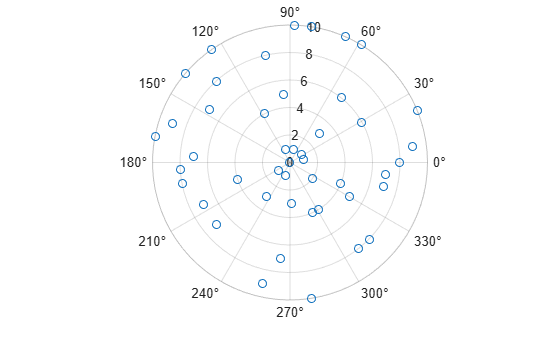You can also plot multiple variables at the same time. For example, plot two sets of radius values by specifying the rvar argument as the cell array {'R1','R2'}. Then add a legend. The legend labels match the variable names.

polarscatter(tbl,'Th',{'R1','R2'})
legendSince R2021b

One way to plot data from a table and customize the colors and marker sizes is to set the ColorVariable and SizeData properties. You can set these properties as name-value arguments when you call the polarscatter function, or you can set them on the Scatter object later.

For example, create a table with three variables of random numbers, and plot the Th and R variables with filled markers. Vary the marker colors by specifying the ColorVariable name-value argument. Return the Scatter object as s, so you can set other properties later.

Th = linspace(0,2*pi,50)';
R = randi([0 10],50,1);
Colors = rand(50,1);
tbl = table(Th,R,Colors);
s = polarscatter(tbl,'Th','R','filled','ColorVariable','Colors');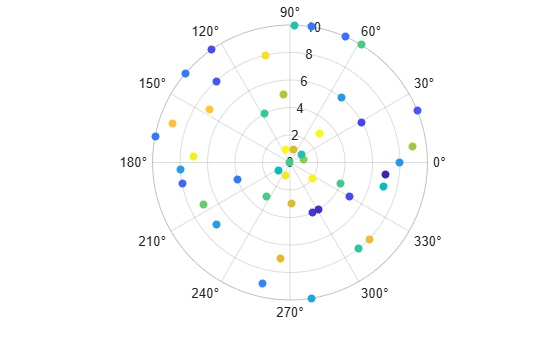Change the marker sizes to 100 points by setting the SizeData property.

s.SizeData = 100;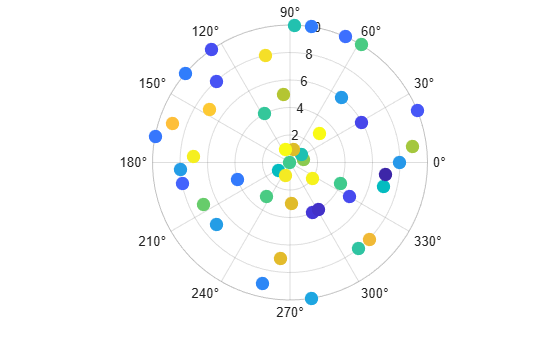Input Arguments

collapse all

Theta values, specified as a scalar, vector, or matrix. The ThetaData property of the scatter object stores the theta values.

The size and shape of theta depends on the shape of your data. This table describes the most common situations.

Type of PlotHow to Specify Coordinates
Single point

Specify theta and rho as scalars. For example:

polarscatter(pi/2,0.5)

One set of points

Specify theta and rho as any combination of row or column vectors of the same length. For example:

polarscatter([0 pi/4 pi/2],[1; 2; 3])

Multiple sets of points that are different colors

If all the sets share the same theta or rho values, specify the shared coordinates as a vector and the other coordinates as a matrix. The length of the vector must match one of the dimensions of the matrix. For example:

polarscatter([0 pi/4 pi/2],[4 5 6; 7 8 9])
If the matrix is square, polarscatter plots a separate set of points for each column in the matrix.

Alternatively, specify theta and rho as matrices of equal size. In this case, polarscatter plots each column of theta against the corresponding column of rho. For example:

polarscatter([0 pi/6 pi/2; pi/8 pi/4 pi],[1 2 3; 4 5 6])

Data Types: single | double | int8 | int16 | int32 | int64 | uint8 | uint16 | uint32 | uint64

Rho values, specified as a scalar, vector, or matrix. The RData property of the scatter object stores the rho values.

The size and shape of rho depends on the shape of your data. This table describes the most common situations.

Type of PlotHow to Specify Coordinates
Single point

Specify theta and rho as scalars. For example:

polarscatter(pi/2,0.5)

One set of points

Specify theta and rho as any combination of row or column vectors of the same length. For example:

polarscatter([0 pi/4 pi/2],[1; 2; 3])

Multiple sets of points that are different colors

If all the sets share the same theta or rho values, specify the shared coordinates as a vector and the other coordinates as a matrix. The length of the vector must match one of the dimensions of the matrix. For example:

polarscatter([0 pi/4 pi/2],[4 5 6; 7 8 9])
If the matrix is square, polarscatter plots a separate set of points for each column in the matrix.

Alternatively, specify theta and rho as matrices of equal size. In this case, polarscatter plots each column of theta against the corresponding column of rho. For example:

polarscatter([0 pi/6 pi/2; pi/8 pi/4 pi],[1 2 3; 4 5 6])

Data Types: single | double | int8 | int16 | int32 | int64 | uint8 | uint16 | uint32 | uint64

Marker size, specified as a numeric scalar, vector, matrix, or empty array ([]). The size controls the area of each marker in points squared. An empty array specifies the default size of 36 points. The way you specify the size depends on how you specify theta and rho, and how you want the plot to look. This table describes the most common situations.

Desired Marker Sizestheta and rho szExample

Same size for all points

Any valid combination of vectors or matrices described for theta and rho.

Scalar

Specify theta as a vector, rho as a matrix, and sz as a scalar.

th = [0 pi/6 pi/2];
r = [1 2; 2 4; 5 6];
polarscatter(th,r,100)

Different size for each point

Vectors of the same length

• A vector with the same length as theta and rho.

• A matrix with at least one dimension that matches the lengths of theta and rho. Specifying a matrix is useful for displaying multiple markers with different sizes at each (th,r) location.

Specify theta, rho, and sz as vectors.

th = [0 pi/6 pi/2];
r = [1; 2; 3];
sz = [50 500 100];
polarscatter(th,r,sz)

Specify theta and rho as vectors and sz as a matrix.

th = [0 pi/6 pi/2];
r = [1; 2; 3];
sz = [50 500 100; 300 1000 200];
polarscatter(th,r,sz)

Different size for each point

At least one of x or y is a matrix for plotting multiple data sets

• A vector with the same number of elements as there are points in each data set.

• A matrix that has the same size as the theta or rho matrix.

Specify theta as a vector, rho as a matrix, and sz as vector.

th = [0 pi/6 pi/2];
r = [1 2; 2 4; 5 6];
sz = [50 500 1000];
polarscatter(th,r,sz)

Specify theta as a vector, rho as a matrix, and sz as a matrix the same size as rho.

th = [0 pi/6 pi/2];
r = [1 2; 2 4; 5 6];
sz = [50 500; 1000 2000; 100 300];
polarscatter(th,r,sz)

Data Types: single | double | int8 | int16 | int32 | int64 | uint8 | uint16 | uint32 | uint64

Marker color, specified as a color name, RGB triplet, matrix of RGB triplets, or a vector of colormap indices.

• Color name — A color name such as 'red', or a short name such as 'r'.

• RGB triplet — A three-element row vector whose elements specify the intensities of the red, green, and blue components of the color. The intensities must be in the range [0,1]; for example, [0.4 0.6 0.7]. RGB triplets are useful for creating custom colors.

• Matrix of RGB triplets — A three-column matrix in which each row is an RGB triplet.

• Vector of colormap indices — A vector of numeric values that is the same length as the theta and rho vectors.

The way you specify the color depends on the desired color scheme and whether you are plotting one set of coordinates or multiple sets of coordinates. This table describes the most common situations.

Color SchemeHow to Specify the ColorExample

Use one color for all the points.

Specify a color name or a short name from the table below, or specify one RGB triplet.

Plot one set of points, and specify the color as 'red'.

th = [0 pi/6 pi/2];
r = [1 2 3];
c = 'red';
polarscatter(th,r,[],c)

Plot two sets of points, and specify the color as red using an RGB triplet.

th = [0 pi/6 pi/2];
r = [1 2; 2 4; 5 6];
c = [1 0 0];
polarscatter(th,r,[],c)

Assign different colors to each point using a colormap.

Specify a row or column vector of numbers. The numbers index into the current colormap array. The smallest value maps to the first row in the colormap, and the largest value maps to the last row. The intermediate values map linearly to the intermediate rows.

If your plot has three points, specify a column vector to ensure the values are interpreted as colormap indices.

You can use this method only when theta, rho, and sz are all vectors.

Create a vector c that specifies four colormap indices. Plot four points using the colors from the current colormap. Then, change the colormap to winter.

th = [0 pi/6 pi/2 2*pi/3];
r = [1 2 3 4];
c = [1 2 3 4];
polarscatter(th,r,[],c)
colormap(gca,'winter')

Create a custom color for each point.

Specify an m-by-3 matrix of RGB triplets, where m is the number of points in the plot.

You can use this method only when theta, rho, and sz are all vectors.

Create a matrix c that specifies the RGB triplets of four colors. Then create plot four points using those colors.

th = [0 pi/6 pi/2 2*pi/3];
r = [1 2 3 4];
c = [1 0 0; 0 1 0; 0 0 1; 0 0 0];
polarscatter(th,r,[],c)

Create a different color for each data set.

Specify an n-by-3 matrix of RGB triplets, where n is the number of data sets.

You can use this method only when at least one of theta, rho, or sz is a matrix.

Create a matrix c that contains two RGB triplets. Then plot two data sets using those colors.

th = [0 pi/6 pi/2];
r = [1 2; 2 4; 5 6];
c = [1 0 0; 0 0 1];
polarscatter(th,r,[],c)

Color Names and RGB Triplets for Common Colors

Color NameShort NameRGB TripletHexadecimal Color CodeAppearance
'red''r'[1 0 0]'#FF0000''green''g'[0 1 0]'#00FF00''blue''b'[0 0 1]'#0000FF''cyan' 'c'[0 1 1]'#00FFFF''magenta''m'[1 0 1]'#FF00FF''yellow''y'[1 1 0]'#FFFF00''black''k'[0 0 0]'#000000''white''w'[1 1 1]'#FFFFFF'Here are the RGB triplets and hexadecimal color codes for the default colors MATLAB® uses in many types of plots.

[0 0.4470 0.7410]'#0072BD'[0.8500 0.3250 0.0980]'#D95319'[0.9290 0.6940 0.1250]'#EDB120'[0.4940 0.1840 0.5560]'#7E2F8E'[0.4660 0.6740 0.1880]'#77AC30'[0.3010 0.7450 0.9330]'#4DBEEE'[0.6350 0.0780 0.1840]'#A2142F'Data Types: single | double | int8 | int16 | int32 | int64 | uint8 | uint16 | uint32 | uint64 | char | string

Marker symbol, specified as one of the marker symbols listed in this table.

MarkerDescriptionResulting Marker
'o'Circle'+'Plus sign'*'Asterisk'.'Point'x'Cross'_'Horizontal line'|'Vertical line's'Square'd'Diamond'^'Upward-pointing triangle'v'Downward-pointing triangle'>'Right-pointing triangle'<'Left-pointing triangle'p'Pentagram'h'HexagramThe Marker property of the scatter object stores the marker symbol.

Source table containing the data to plot. Specify this argument as a table or a timetable.

Table variables containing the theta values, specified as one or more table variable indices.

Specifying Table Indices

Use any of the following indexing schemes to specify the desired variable or variables.

Indexing SchemeExamples

Variable names:

• A character vector or a string scalar.

• A cell array or string vector.

• 'A' or "A" — A variable called A

• {'A','B'} or ["A","B"] — Two variables called A and B

Variable numbers:

• An index number that refers to the location of a variable in the table.

• A vector of numbers.

• 3 — The third variable from the table

• [2 3] — The second and third variables from the table

Logical vector:

• An n-element vector logical vector, where logical 1 (true) values indicate the desired variables.

• Optionally, omit the trailing false values after the last true value.

• [false false true false] — The third variable from an m-by-4 table

• [false false true] — The third variable from any size table

• [false true true] — The second and third variables from any size table

Variable type:

• A vartype command that selects table variables of a specified type.

• vartype('categorical') — All the variables containing categorical values

The table variables you specify can contain any numeric data type.

To plot one data set, specify one variable for thetavar and one variable for rhovar. For example, create a table with three variables. Plot the Th and R1 variables.

% Create a table of random numbers
Th = linspace(0,2*pi,10)';
R1 = randi([0 10],10,1);
R2 = randi([20 30],10,1);
tbl = table(Th,R1,R2);

% Create polar scatter chart
polarscatter(tbl,'Th','R1')

To plot multiple data sets together, specify multiple variables for thetavar, rhovar, or both. If you specify multiple variables for both arguments, the number of variables for each argument must be the same.

For example, plot the Th variable on the theta-axis and the R1 and R2 variables on the r-axis.

polarscatter(tbl,'Th',{'R1','R2'})

You can also use different indexing schemes for the table variables. For example, specify thetavar as a variable name and rhovar as an index number.

polarscatter(tbl,'Th',2)

Table variables containing the rho values, specified as one or more table variable indices.

Specifying Table Indices

Use any of the following indexing schemes to specify the desired variable or variables.

Indexing SchemeExamples

Variable names:

• A character vector or a string scalar.

• A cell array or string vector.

• 'A' or "A" — A variable called A

• {'A','B'} or ["A","B"] — Two variables called A and B

Variable numbers:

• An index number that refers to the location of a variable in the table.

• A vector of numbers.

• 3 — The third variable from the table

• [2 3] — The second and third variables from the table

Logical vector:

• An n-element vector logical vector, where logical 1 (true) values indicate the desired variables.

• Optionally, omit the trailing false values after the last true value.

• [false false true false] — The third variable from an m-by-4 table

• [false false true] — The third variable from any size table

• [false true true] — The second and third variables from any size table

Variable type:

• A vartype command that selects table variables of a specified type.

• vartype('categorical') — All the variables containing categorical values

The table variables you specify can contain any numeric data type.

To plot one data set, specify one variable for thetavar and one variable for rhovar. For example, create a table with three variables. Plot the Th and R1 variables.

% Create a table of random numbers
Th = linspace(0,2*pi,10)';
R1 = randi([0 10],10,1);
R2 = randi([20 30],10,1);
tbl = table(Th,R1,R2);

% Create polar scatter chart
polarscatter(tbl,'Th','R1')

To plot multiple data sets together, specify multiple variables for thetavar, rhovar, or both. If you specify multiple variables for both arguments, the number of variables for each argument must be the same.

For example, plot the Th variable on the theta-axis and the R1 and R2 variables on the r-axis.

polarscatter(tbl,'Th',{'R1','R2'})

You can also use different indexing schemes for the table variables. For example, specify thetavar as a variable name and rhovar as an index number.

polarscatter(tbl,'Th',2)

PolarAxes object. If you do not specify the polar axes, then polarscatter uses the current axes. polarscatter does not support plotting into Cartesian axes.

Name-Value Arguments

Specify optional comma-separated pairs of Name,Value arguments. Name is the argument name and Value is the corresponding value. Name must appear inside quotes. You can specify several name and value pair arguments in any order as Name1,Value1,...,NameN,ValueN.

Example: polarscatter(th,r,'filled','MarkerFaceAlpha',.5) creates filled, semi-transparent markers.

The scatter object properties listed here are only a subset. For a complete list, see Scatter Properties.

Marker face transparency, specified as a scalar in the range [0,1]. A value of 1 is opaque and 0 is transparent. Values between 0 and 1 are semi-transparent.

Marker outline color, specified 'flat', an RGB triplet, a hexadecimal color code, a color name, or a short name. The default value of 'flat' uses colors from the CData property.

For a custom color, specify an RGB triplet or a hexadecimal color code.

• An RGB triplet is a three-element row vector whose elements specify the intensities of the red, green, and blue components of the color. The intensities must be in the range [0,1]; for example, [0.4 0.6 0.7].

• A hexadecimal color code is a character vector or a string scalar that starts with a hash symbol (#) followed by three or six hexadecimal digits, which can range from 0 to F. The values are not case sensitive. Thus, the color codes '#FF8800', '#ff8800', '#F80', and '#f80' are equivalent.

Alternatively, you can specify some common colors by name. This table lists the named color options, the equivalent RGB triplets, and hexadecimal color codes.

Color NameShort NameRGB TripletHexadecimal Color CodeAppearance
'red''r'[1 0 0]'#FF0000''green''g'[0 1 0]'#00FF00''blue''b'[0 0 1]'#0000FF''cyan' 'c'[0 1 1]'#00FFFF''magenta''m'[1 0 1]'#FF00FF''yellow''y'[1 1 0]'#FFFF00''black''k'[0 0 0]'#000000''white''w'[1 1 1]'#FFFFFF''none'Not applicableNot applicableNot applicableNo color

Here are the RGB triplets and hexadecimal color codes for the default colors MATLAB uses in many types of plots.

[0 0.4470 0.7410]'#0072BD'[0.8500 0.3250 0.0980]'#D95319'[0.9290 0.6940 0.1250]'#EDB120'[0.4940 0.1840 0.5560]'#7E2F8E'[0.4660 0.6740 0.1880]'#77AC30'[0.3010 0.7450 0.9330]'#4DBEEE'[0.6350 0.0780 0.1840]'#A2142F'Example: [0.5 0.5 0.5]

Example: 'blue'

Example: '#D2F9A7'

Marker fill color, specified as 'flat', 'auto', an RGB triplet, a hexadecimal color code, a color name, or a short name. The 'flat' option uses the CData values. The 'auto' option uses the same color as the Color property for the axes.

For a custom color, specify an RGB triplet or a hexadecimal color code.

• An RGB triplet is a three-element row vector whose elements specify the intensities of the red, green, and blue components of the color. The intensities must be in the range [0,1]; for example, [0.4 0.6 0.7].

• A hexadecimal color code is a character vector or a string scalar that starts with a hash symbol (#) followed by three or six hexadecimal digits, which can range from 0 to F. The values are not case sensitive. Thus, the color codes '#FF8800', '#ff8800', '#F80', and '#f80' are equivalent.

Alternatively, you can specify some common colors by name. This table lists the named color options, the equivalent RGB triplets, and hexadecimal color codes.

Color NameShort NameRGB TripletHexadecimal Color CodeAppearance
'red''r'[1 0 0]'#FF0000''green''g'[0 1 0]'#00FF00''blue''b'[0 0 1]'#0000FF''cyan' 'c'[0 1 1]'#00FFFF''magenta''m'[1 0 1]'#FF00FF''yellow''y'[1 1 0]'#FFFF00''black''k'[0 0 0]'#000000''white''w'[1 1 1]'#FFFFFF''none'Not applicableNot applicableNot applicableNo color

Here are the RGB triplets and hexadecimal color codes for the default colors MATLAB uses in many types of plots.

[0 0.4470 0.7410]'#0072BD'[0.8500 0.3250 0.0980]'#D95319'[0.9290 0.6940 0.1250]'#EDB120'[0.4940 0.1840 0.5560]'#7E2F8E'[0.4660 0.6740 0.1880]'#77AC30'[0.3010 0.7450 0.9330]'#4DBEEE'[0.6350 0.0780 0.1840]'#A2142F'Example: [0.3 0.2 0.1]

Example: 'green'

Example: '#D2F9A7'

Width of marker edge, specified as a positive value in point units.

Example: 0.75

Table variable containing the color data, specified as a variable index into the source table.

Specifying the Table Index

Use any of the following indexing schemes to specify the desired variable.

Indexing SchemeExamples

Variable name:

A character vector or a string scalar.

• 'A' or "A" — A variable called A

Variable number:

An index number that refers to the location of a variable in the table.

• 3 — The third variable from the table

Logical vector:

• An n-element vector logical vector, where logical 1 (true) values indicate the desired variable.

• Optionally, omit the trailing false values after the true value.

• [false false true false] — The third variable from an m-by-4 table

• [false false true] — The third variable from any size table

Variable type:

A vartype command that selects table variables of a specified type.

• vartype('double') — The variable containing double values

Specifying Color Data

Specifying the ColorVariable property controls the colors of the markers. The data in the variable controls the marker fill color when the MarkerFaceColor property is set to 'flat'. The data can also control the marker outline color, when the MarkerEdgeColor is set to 'flat'.

The table variable you specify can contain values of any numeric type. The values can be in either of the following forms:

• A column of numbers that linearly map into the current colormap.

• A three-column array of RGB triplets. RGB triplets are three-element vectors whose values specify the intensities of the red, green, and blue components of specific colors. The intensities must be in the range [0,1]. For example, [0.5 0.7 1] specifies a shade of light blue.

When you set the ColorVariable property, MATLAB updates the CData property.